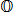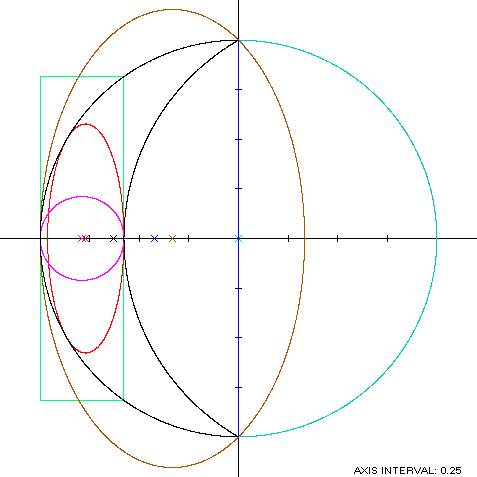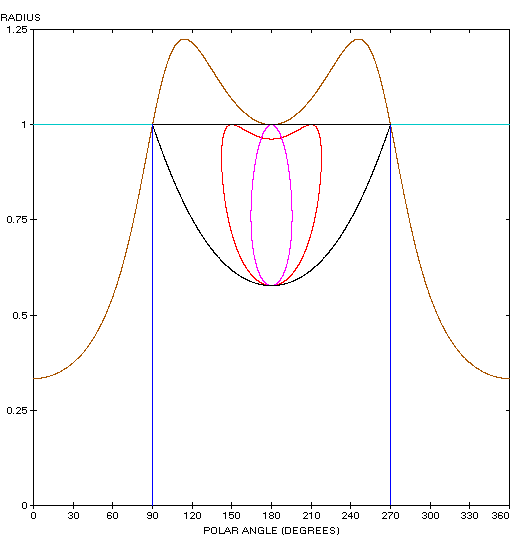Lune
LUNE

Balmoral Software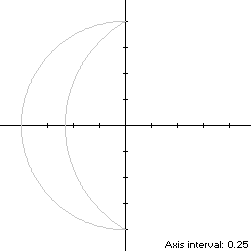The lune is a crescent-shaped, x-symmetric closed curve S bounded by the arcs of two circles of different radii. Here we have taken the cusps of S at the endpoints of a diameter of a unit circle, and 2π/3 as the angle subtended in a larger circle by that line segment: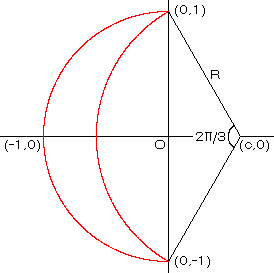To find the radius R and center abscissa c of the larger circle, we have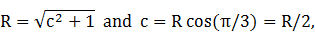which has solutionSo that S is traversed in a clockwise direction starting from its left edge (-1,0) at t = 0, its parametric equations are: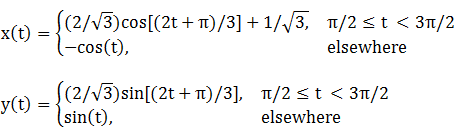The abscissa maxima of the lune are at the two cusps (0,±1), so it is non-convex by the multiple local extrema test. The ordinate extrema are also at the cusps, so the width x height of the lune's bounding rectangle is 1 x 2. The lune crosses the x-axis at -1 and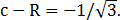### Convex Hull

The convex hull of the lune is created simply by connecting its two cusps with a vertical line segment of length 2, as shown in blue in the left diagram below. The perimeter of the convex hull is half the circumference of a unit circle plus the line segment, or π + 2. The area of the convex hull is that of a unit semicircle, or π/2. The centroid abscissa of the convex hull is that of a unit semicircle centered at the origin and in the left half-plane, or -4/(3π).

### Metrics

The perimeter of the lune is the semicircumference of a unit circle plus the arc length subtending 2π/3 on a larger circle of radius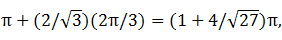so the perimeter of the convex hull is about about 8% shorter than that of the lune itself.

The portion of the unit semicircle that is not part of the lune is the segment of the larger circle, which has areaThe centroid abscissa of this segment is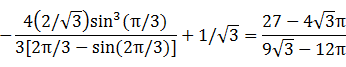The lune metrics can be summarized as follows:
 Area Centroid abscissa Product Region Convex hull π/2 -4/(3π) -2/3 Segment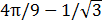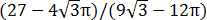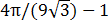Lune (by subtraction)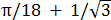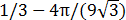The convex hull has a little more than twice the area of the lune. The centroid abscissa of the lune is is the weighted averageThe bounding rectangle for inconics is delimited by the upper x-intercept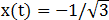occurring when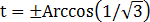and the corresponding outer ordinates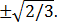### Incircle

A candidate incircle is centered on the x-axis between the axis crossings of S, and so has radius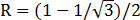and center abscissa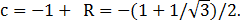For verification, we have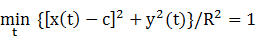### Inellipse

Usingin Lemma E,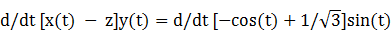has a zero at t* = π/6. The corresponding coordinates are: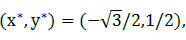so the inellipse dimensions are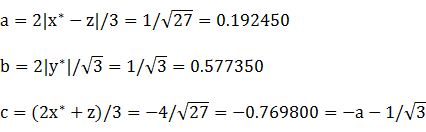For verification,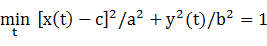### Circumellipse

The extreme points of the lune create an x-symmetric isosceles triangle with vertices (-1,0) and (0,±1). Using h = -1 and w = 2 in Lemma TE, a candidate for the circumellipse has parameters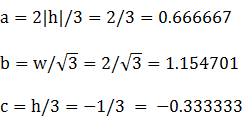For verification,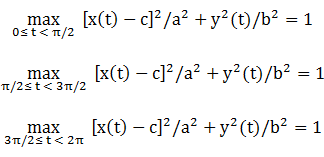### Circumcircle

Since the lune is a portion of a unit circle centered at the origin, its circumcircle is that circle.

### Summary Table

 Perimeter Area Centroid Figure Parameters Incircle R =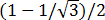1.327793 0.140298 (-0.788675,0) Inellipse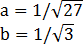2.572074 0.349066 (-0.769800,0) Lune Width: 1Height: 2 5.559992 0.751883 (-0.628821,0) Convex hull 5.141593 1.570796 (-0.424413,0) Circumellipse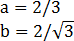5.825171 2.418401 (-0.333333,0) Circumcircle R = 1 6.283185 3.141593